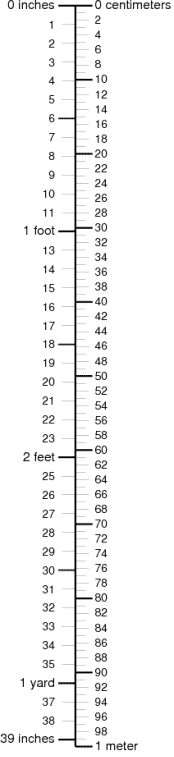•• # Centimeters Inches

Both centimeters and inches are units of length in themetric and imperial system respectively. You don’t usually meddle with the conversion of one unit of measure to the other unless you’re working on a project or you’re doing something that will require you to do the said calculation. Otherwise, we simply would be happy to live with either unit since they denote the very same thing.

### The Centimeter

Given the meaning behind its name, this unit of measure is a hundredth of a single meter. This is actually one of the SI base units to measure length. It is the equivalent of 0.01 meter. This unit of measure has been found to be quite useful. It can be used to measure smaller lengths and is also used to denote the height of individuals.

One of its uses is for the reporting of the amount of rainfall received in a given area using a rain gauge. If you look at a map you’ll notice that this unit of measurement is also used to convert map scales into the scales or actual sizes in the real world. It is also used to measure capacitance in the CGS system. It is denoted by the symbol ‘cm’ whenever it is used.

### The Inch

The symbols used to denote inches are ‘in’ and a double prime (i.e. “). Like the centimeter, this is also a unit of measuring length. It is used in a number of systems of measurement including the US customary units and the Imperial units of measurement. It is quite a common unit used in many parts of the world including Canada, the United Kingdom, and the United States. Other commonwealth nations also make use of this unit when measuring lengths.

### The Need for Conversion

The need to convert from one of these units to the other may sometimes arise. For instance, some folks are used to expressing their height in feet and inches. When another person’s height is expressed in centimeters it is natural to try and figure out what the equivalent of such height is.

### Doing the Math

Doing the calculations yourself is pretty easy as long as you know the base equivalents. All you need to do is a little bit of math then you’re good to go. However, if you’re not up to crunching numbers no matter how small you can either consult an online unit converter or just look up a conversion table that shows both of these units of measurement. In case you still want to exercise your brain cells then just remember that an inch is the equivalent of 2.54 centimeters.